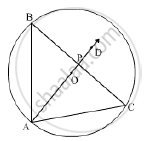Share

Two Chords Ab and Ac of a Circle Are Equal. Prove that the Centre of the Circle Lies on the Bisector of Angle Bac. - ICSE Class 10 - Mathematics

ConceptChord Properties - the Perpendicular to a Chord from the Center Bisects the Chord (Without Proof)

Question

Two chords AB and AC of a circle are equal. Prove that the centre of the circle lies on the bisector of angle BAC.

SolutionGiven: AB and AC are two equal chords of C (O, r).
To prove: Centre, O lies on the bisector of ∠BAC.
Construction: Join BC. Let the bisector of ∠BAC intersects BC in P.
Proof:
In ΔAPB and ΔAPC,
AB = AC (Given)
∠ BAP = ∠CAP (Given)
AP = AP (Common)
∴ Δ APB  ≅  ΔAPC (SAS congruence criterion)
⇒ BP = CP and ∠APB = ∠APC (CPCT)
∠APB + ∠APC = 180° (Linear pair)
⇒  2 ∠APB = 180° (∠APB = ∠APC)
⇒  ∠APB = 90°
Now, BP = CP and ∠APB = 90°
∴ AP is the perpendicular bisector of chord BC.
⇒ AP passes through the centre, O of the circle.

Is there an error in this question or solution?

APPEARS IN

Solution Two Chords Ab and Ac of a Circle Are Equal. Prove that the Centre of the Circle Lies on the Bisector of Angle Bac. Concept: Chord Properties - the Perpendicular to a Chord from the Center Bisects the Chord (Without Proof).
S